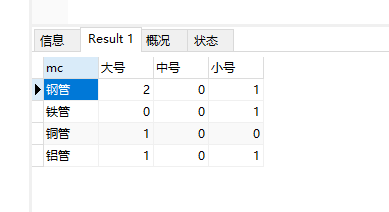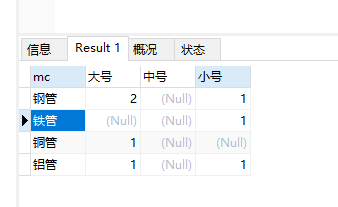# mysql如何分类统计数量

2020-03-30 17:04:37

```id    mc            xh
1    钢管          大号
2    铜管          大号
3    铁管          小号
4    铝管          中号
5    钢管          小号```

1.在mysql中建立一个测试数据表

```CREATE TABLE `tb_test_count` (
`id` bigint(20) NOT NULL,
`mc` varchar(255) CHARACTER SET utf8mb4 DEFAULT NULL,
`xh` varchar(255) CHARACTER SET utf8mb4 DEFAULT NULL,
PRIMARY KEY (`id`)
) ENGINE=InnoDB DEFAULT CHARSET=latin1;```

2.插入一批数据

```INSERT INTO tb_test_count VALUES(1,'钢管','大号');
INSERT INTO tb_test_count VALUES(2,'铜管','大号');
INSERT INTO tb_test_count VALUES(3,'铁管','小号');
INSERT INTO tb_test_count VALUES(4,'铝管','大号');
INSERT INTO tb_test_count VALUES(5,'铝管','小号');
INSERT INTO tb_test_count VALUES(6,'钢管','大号');
INSERT INTO tb_test_count VALUES(7,'钢管','小号');```

3.查询统计的SQL语句

```select mc,count(case when xh='大号' then 1 end) as 大号,
count(case when xh='中号' then 1 end) as 中号,
count(case when xh='小号' then 1 end) as 小号
from tb_test_count
group by mc```

4.查询结果截图如下：5.如果使用sum方法查询则(当数据一条都不存在的时候查询出来的结果是null)

```select mc,sum(case when xh='大号' then 1 end) as 大号,
sum(case when xh='中号' then 1 end) as 中号,
sum(case when xh='小号' then 1 end) as 小号
from tb_test_count
group by mc```